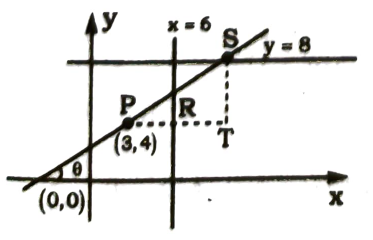Equation of line in Straight lines
Question

# A line not parallel to the axes drawn through the point P(3,4) and inclined at an angle  $\theta$ to the positive direction of x-axis. If the lines meets the line x=6 and y=8 at R and S respectively then

Easy
Solution

##$\mathrm{cos}\mathrm{\theta }=\frac{3}{\mathrm{PR}},\mathrm{sin}\mathrm{\theta }=\frac{4}{\mathrm{PS}}$

Get Instant Solutions